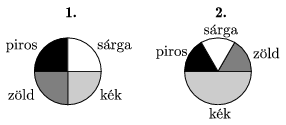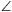Mathematical and Physical Journal
for High Schools
Issued by the MATFUND Foundation
 Already signed up? New to KöMaL?

# KöMaL Problems in Mathematics, February 2006

Show/hide problems of signs:## Problems with sign 'K'

Deadline expired on March 10, 2006.

K. 73. ABCD is a square of 5-cm sides. An equilateral triangle CDE is drawn outwards to the side CD. a) Find the angles of the triangle ABE. b) What is the radius of the circle that passes through all three vertices of triangle ABE?

(6 pont)

solution (in Hungarian), statistics

K. 74. The Figure shows two paint boxes. Ann, Bob, Clare and Diana are mixing paints for decorating eggs as follows: Wit eyes closed, they dip their paintbrush twice in a row in one compartment of one box, and then apply the colour obtained in this way to the egg. Ann dips her brush in the first box both times, Bob uses the second box both times, Clare dips her brush first in the first box and then in the second box, and Diana dips it in the first one and then in the second one. Which of them has the lowest chance of getting a purple colour (red + blue)?(6 pont)

solution (in Hungarian), statistics

K. 75. The point K lies on each of the circles of radius r centred at the points K1, K2, K3. The other common points of two of the three circles are P, Q, and R. Show that the triangles K1K2K3 and PQR are congruent.

(6 pont)

solution (in Hungarian), statistics

K. 76. 2006 small circles are placed in a large circle, so that each touches the next one in a row on the outside, the centres of the small circles all lie on the same diameter of the large circle, and the sum of the diameters of the small circles equals the diameter of the large circle. Compare the total perimeter of the small circles and the perimeter of the large circle.

(6 pont)

solution (in Hungarian), statistics

K. 77. The Silly family bought a tape measure that can be hung on the wall for measuring heights of people. One end of the tape reads 80 cm'' and the other end reads 180 cm'' (If he person to be measured stands on the floor, the tape can measure heights between 80 and 180 cm if properly used.) The Sillies hung the tape in a vertical position, but its lower end was not fixed at the appropriate height.To make it even worse, it also turned out that even the centimetre scale on the tape was wrong: the divisions of were not actually 1 cm apart. Thus the head of the 130-cm-tall Pete reached up to the 120-cm mark while the tape read 145 cm for his 150-cm-tall sister. What is the maximum height of a person that can be measured with this measuring tape with improper scale hung improperly on the wall?

(6 pont)

solution (in Hungarian), statistics

K. 78. If the measure of the surface area of a cuboid (in unit squares) is subtracted from the sum of the measures of its edges (in length units), and the measure of the volume of the cuboid (in unit cubes) is added to the number obtained, the result will be 8. Prove that the measure of one of the edges is 2.

(6 pont)

solution (in Hungarian), statistics## Problems with sign 'C'

Deadline expired on March 16, 2006.

C. 840. The values of 10 banknotes add up to 5000 forints (HUF). What banknotes may this sum consist of? (The Hungarian bank notes are: 200 HUF, 500 HUF, 1000 HUF, 2000 HUF, 5000 HUF, 10 000 HUF, 20 000 HUF.)

(5 pont)

solution (in Hungarian), statistics

C. 841. Paul the postman was delivering letters in a long street. First he walked down the street on one side (where the odd-numbered blocks were) and then he walked back on the other side (delivering the letters to the even-numbered blocks). On the odd side he spent one third as much time standing in front of the letter boxes as he spent by walking back on the even side. On his way back on the even side his total standing time in front of the letter boxes was one third as much as his time in motion down the street on the odd side. Finally, it turned out that delivering the letters took the same time on each side. Find the ratio of his average walking speed (not counting the stops) on the odd side to his average walking speed back on the even side.

(5 pont)

solution (in Hungarian), statistics

C. 842. We built a large solid cube out of more than ten wooden cubes of unit edge, each. Then we painted all faces of the large cube. Is it possible that the number of unit cubes that have some faces painted is a multiple of the number of the unpainted ones?

(5 pont)

solution (in Hungarian), statistics

C. 843. In a triangle ABC,BAC=45o. Let P denote the point of side AC for which AP:PC=1:2. Given thatABP=15o findACB.

(5 pont)

solution (in Hungarian), statistics

C. 844. The perimeter of the axial section of a cylinder is 90 cm. What is the maximum possible volume of the cylinder?

(5 pont)

solution (in Hungarian), statistics## Problems with sign 'B'

Deadline expired on March 16, 2006.

B. 3882. BAD and ACE are the regular triangles drawn over two sides of a triangle ABC on the outside. Show that the reflection of the line BE about the line CD passes through the point A.

(3 pont)

solution (in Hungarian), statistics

B. 3883. Prove that if the difference of the integers a, b is divisible by 100, then a100-b100 is divisible by 10 000.

(3 pont)

solution (in Hungarian), statistics

B. 3884. Three unit circles all pass through the point P. Their other intersections are A, B and C. Find the radius of the circumscribed circle of the triangle ABC.

(4 pont)

solution (in Hungarian), statistics

B. 3885. The radius of the iscribed circle of a triangle is r, the radius of its circumcircle is R, and one of the angles is. Given thatshow that the triangle is isosceles.

(4 pont)

solution (in Hungarian), statistics

B. 3886. The diagonals of a cyclic quadrilateral ABCD are perpendicular. A perpendicular is dropped from the intersection of the diagonals onto the line AB. In what ratio does the perpendicular divide the side CD?

(4 pont)

solution (in Hungarian), statistics

B. 3887. We built a large solid cube out of more than ten wooden cubes of unit edge. Then we painted all faces of the large cube. Is it possible that the number of unit cubes that have some faces is a factor of the number of unpainted ones?

(4 pont)

solution (in Hungarian), statistics

B. 3888. For what integers m>1 is there an ordering a1,a2,...,am of the numbers 1,2,...,m, such that the sums a1,a1+a2,...,a1+a2+...+am leave different remainders when divided by m?

(5 pont)

solution (in Hungarian), statistics

B. 3889. Here is a magician's trick that I have seen: He asked one of the spectators to choose 5 cards at random from a deck of 52 French cards and hand them to his assistant. (The assistant was a permanent partner of the magician, so they could have talked before.) The assistant looked at the 5 cards, and handed four of them over to the magician one by one. Then the magician, without receiving any further information, named the fifth card. After the trick, the magician tried to convince mathematically minded spectators as follows:

My assistant showed me four cards, and those four cards may be any four of the deck, therefore the only way he could convey information to me was through the order of the four cards he showed to me. Since, as you know, there are 4!=24 possible orders of four cards, and the fifth card could be any of 52-4=48, exactly 1 bit of information was missing. It is the guessing of this bit that needs a miracle.''

Does the trick really need supernatural powers?

Suggested by M. Gáspár Előd, physicist

(5 pont)

solution (in Hungarian), statistics

B. 3890. There are 200 participants at an international conference. Each of them speaks at least 4 languages, and in any three of them there are two who speak a language in common. Prove that there is a language that at least 26 participants speak.

(4 pont)

solution (in Hungarian), statistics

B. 3891. Given that x and y are rational numbers, such that x5+y5=2x2y2. Prove that 1-xy is the square of a rational number.

(5 pont)

solution (in Hungarian), statistics## Problems with sign 'A'

Deadline expired on March 16, 2006.

A. 392. The inradius of a tetrahedron is r. There exists three spheres which touch the four face planes such that all touching points are outside the surface of the tetrahedron; the radii of those spheres are r1, r2 and r3. Show that r1+r2+r3>9r.

(5 pont)

solution (in Hungarian), statistics

A. 393. Prove that if n>1 is an integer such that 3n+4n is divisible by n then n is divisible by 7.

(5 pont)

solution, statistics

A. 394. Let a1,a2,...,aN be nonnegative reals, not all 0. Prove that there exists a sequence 1=n0<n1<...<nk=N+1 of integers such that

n1an0+n2an1+...+nkank-1<3(a1+a2+...+aN).

(5 pont)

solution, statistics